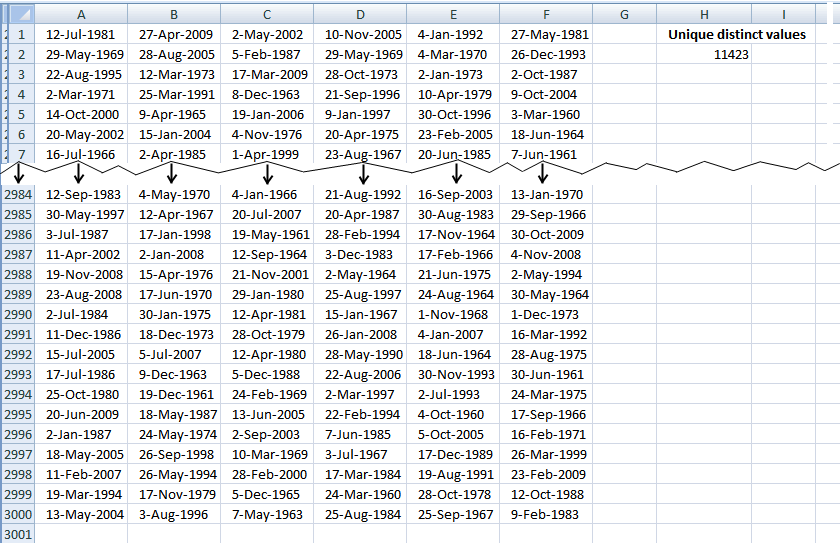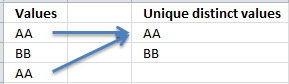Author: Oscar Cronquist Article last updated on January 05, 2019This article describes how to count unique distinct values in list. What is a unique distinct list? Merge all duplicates to one distinct value and you have created a unique distinct list.Formulas are sometimes too slow if you work with a lot of data, this article demonstrates a user defined function you can use, however, I highly recommend using a pivot table for this task:
Count unique distinct values using a pivot table

Formula in cell H2:

=CountUniqueValues(A1:F3000)

### Excel user defined function

```Public Function CountUniqueValues(rng As Variant) As Variant

Dim Test As New Collection
Dim Value As Variant

rng = rng.Value

On Error Resume Next
For Each Value In rng

If Len(Value) > 0 Then Test.Add Value, CStr(Value)

Next Value
On Error GoTo 0

CountUniqueValues = Test.Count

End Function

```